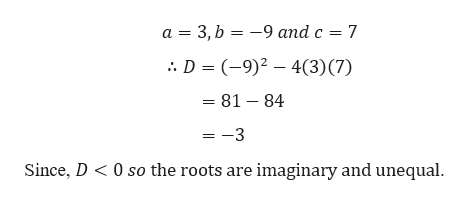# Evaluate the discriminate for the following equation then use it to determine the number of distinct solutions and tell whether they are rational irrational or non real complex numbers 3X squared equals negative 9X + 7

Question

Evaluate the discriminate for the following equation then use it to determine the number of distinct solutions and tell whether they are rational irrational or non real complex numbers

3X squared equals negative 9X + 7

check_circleExpert Solution
Step 1

Given,

Step 2

Here, we h...help_outlineImage Transcriptionclosea 3, b -9 and c 7 D (-9) 43) (7) =81 84 =-3 0 so the roots are imaginary and unequal Since, D fullscreen

### Want to see the full answer?

See Solution

#### Want to see this answer and more?

Solutions are written by subject experts who are available 24/7. Questions are typically answered within 1 hour*

See Solution
*Response times may vary by subject and question
Tagged in

### Polynomials